## B.6 Estimation of TX chain amplitude and frequency response parameters

36.1083GPPEvolved Universal Terrestrial Radio Access (E-UTRA)Release 18Satellite Access Node radio transmission and receptionTS

The equalizer coefficients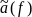and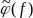are determined as follows:

1. Calculate the complex ratios (amplitude and phase) of the post-FFT acquired signal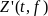and the post-FFT Ideal signal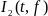, for each reference symbol, over 10 subframes. This process creates a set of complex ratios: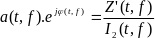Where the post-FFT Ideal signal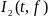is constructed by the measuring equipment according to the relevant TX specifications, using the following parameters: restricted content: i.e. nominal Reference Symbols and the Primary Synchronisation Channel, (all other modulation symbols are set to 0 V), nominal carrier frequency, nominal amplitude and phase for each applicable subcarrier, nominal timing.

2. Perform time averaging at each reference signal subcarrier of the complex ratios, the time-averaging length is 10 subframes. Prior to the averaging of the phases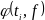an unwrap operation must be performed according to the following definition: The unwrap operation corrects the radian phase angles of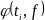by adding multiples of 2*PI when absolute phase jumps between consecutive time instances ti are greater then or equal to the jump tolerance of PI radians. This process creates an average amplitude and phase for each reference signal subcarrier (i.e. every third subcarrier with the exception of the reference subcarrier spacing across the DC subcarrier).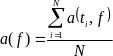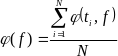Where N is the number of reference symbol time-domain locations ti from Z'(f,t) for each reference signal subcarrier.

3. The equalizer coefficients for amplitude and phase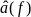and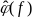at the reference signal subcarriers are obtained by computing the moving average in the frequency domain of the time-averaged reference signal subcarriers, i.e. every third subcarrier. The moving average window size is 19. For reference subcarriers at or near the edge of the channel the window size is reduced accordingly as per figure E.6-1.

4. Perform linear interpolation from the equalizer coefficients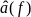and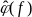to compute coefficients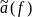,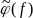for each subcarrier.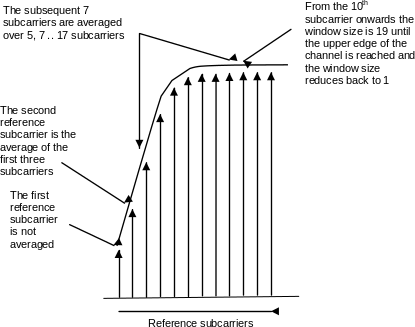Figure E.6-1: Reference subcarrier smoothing in the frequency domain